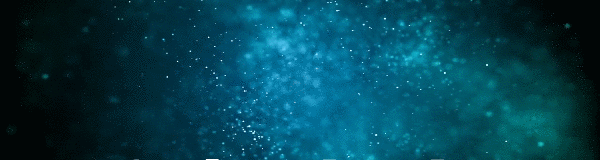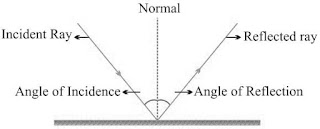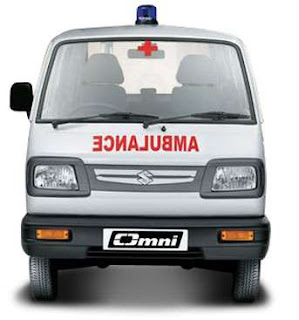--># STD 10 SCIENCE CHAPTER 2 Light – Reflection Class 10th Science MCQ TEST PLAY ONLINE

#### Study Material and Notes of Ch 10 Light – Reflection Class 10th Science

Topics in the Chapter

• Introduction
• Properties of Light
• Reflection
→ Laws of Reflection
→ Virtual and Real image
• Image formed by Plane mirror
→ Characteristics of image formed by Plane mirror
→ Lateral inversion and its application
• Spherical Mirrors
→ Properties of Concave mirror
→ Properties of Convex mirror
→ Common terms for Spherical mirrors
• Rules for making ray diagrams by spherical mirrors
• Ray diagrams for images formed by concave mirror
→ When object is at infinity
→ When object is beyond C
→ When object is at C
→ When object is placed between F and C
→ When object is placed at F
→ When object is between P and F
• Uses of Concave mirror
• Ray diagrams of images formed by convex mirror
→ When object is placed at infinity
→ When object is placed between pole and infinity
• Uses of convex mirror
• Sign convention for reflection by spherical mirror
• Mirror Formula
→ Magnification of Spherical mirrors

Introduction
→ Light is the form of energy that provides sensation of vision.

→ Some common phenomena associated with lights are image formation by mirrors, the twinkling of stars, the beautiful colours of a rainbow, bending of light by a medium and so on.

Properties of Light

→  Electromagnetic wave, so does not require any medium to travel.

→  Light tends to travel in straight line.

→  Light has dual nature i.e. wave as well as particle.

→ Speed of light is maximum in vaccum. Its value is 3 × 108 ms-1.

→ When light falls on a surface, following may happen:
(i) Reflection
(ii) Refraction
(iii) Absorption

Reflection

→ Bouncing back of light when it strikes on a polished surface like mirror.

Laws of Reflection

(i) Angle of incidence is equal to the angle of reflection.

(ii) The incident ray, the reflected ray and the normal at the point of incidence, all lie in the same plane.Virtual and Real image
Image is a point where atleast two light rays actually meet or appear to meet.
 Real Image Virtual Image Formed when light rays actually meet. Formed when light rays appear to meet. Can be obtained on screen. Can’t be obtained on screen. Inverted Erect Example: image formed on cinema screen and formed by concave mirror. Example: image formed by plane mirror or convex mirror.
Image Formed by Plane MirrorCharacteristics of Image formed by Plane Mirror
(i)  Virtual and erect.
(ii) Size of image is equal to the size of object.
(iii) Image is formed as far behind the mirror as the object is in front of it.
(iv) Laterally inverted.
Lateral Inversion: The right side of the object appears left side of the image and vice-versa.
Application of lateral inversion
→ The word AMBULANCE is written in reverse direction so that it can be read correctly in rear view mirror of vehicles going in front of it.